# Introduction to ClustGeo

#### 2021-09-30

The R package ClustGeo implements a Ward-like hierarchical clustering algorithm including spatial/geographical constraints. Two dissimilarity matrices D0 and D1 are inputted, along with a mixing parameter alpha in $$[0,1]$$. The dissimilarities can be non-Euclidean and the weights of the observations can be non-uniform. The first matrix gives the dissimilarities in the “feature space”” and the second matrix gives the dissimilarities in the “constraint space”. The criterion minimized at each stage is a convex combination of the homogeneity criterion calculated with D0 and the homogeneity criterion calculated with D1. The idea is to determine a value of alpha which increases the spatial contiguity without deteriorating too much the quality of the solution based on the variables of interest i.e. those of the feature space. This procedure is illustrated on the estuary dataset available in the package.

## The estuary dataset

The dataset estuary refers to $$n=303$$ French municipalities of Gironde estuary (a south-ouest French region). This dataset contains:

• a data frame dat with the description of the 303 municipalities on 4 socio-economic variables,
• a matrix D.geo with the distances between the town halls of the 303 municipalities,
• an object map of class SpatialPolygonsDataFrame with the map of the municipalities.
library(ClustGeo)
data(estuary)
dat <- estuary$dat head(dat) ## employ.rate.city graduate.rate housing.appart agri.land ## 17015 28.08 17.68 5.15 90.04438 ## 17021 30.42 13.13 4.93 58.51182 ## 17030 25.42 16.28 0.00 95.18404 ## 17034 35.08 9.06 0.00 91.01975 ## 17050 28.23 17.13 2.51 61.71171 ## 17052 22.02 12.66 3.22 61.90798 D.geo <- estuary$D.geo
map <- estuary$map The object map is an object of class SpatialPolygonsDataFrame (of the package sp) and the method plot can be used to visualize the municipalities on the map. # description of 5 municipalities in the map head(map@data[,4:8])  ## NOM_COMM X_CHF_LIEU Y_CHF_LIEU X_CENTROID Y_CENTROID ## 17015 ARCES 3989 65021 3979 65030 ## 17021 ARVERT 3791 65240 3795 65247 ## 17030 BALANZAC 4018 65230 4012 65237 ## 17034 BARZAN 3991 64991 3982 64997 ## 17050 BOIS 4189 64940 4176 64934 ## 17052 BOISREDON 4227 64745 4224 64749 # plot of the municipalities library(sp) ?"SpatialPolygonsDataFrame-class" sp::plot(map, border="grey") # plot method sel <- map$NOM_COMM%in% c("BORDEAUX", "ARCACHON", "ROYAN") # label of 3 municipalities
text(sp::coordinates(map)[sel,],
labels = map$NOM_COMM[sel])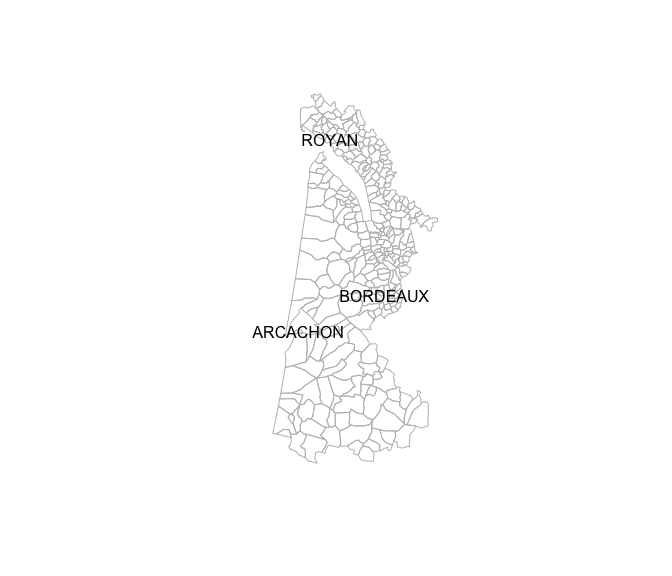# we check that the municipalities in map are the same than those in X identical(as.vector(map$INSEE_COM),rownames(dat))
##  TRUE

## Hierarchical clustering with soft contiguity constraint.

The function hclustgeo implements a Ward-like hierarchical clustering algorithm with soft contiguity constraint. The main arguments of the function are:

• a matrix D0 with the dissimilarities in the “feature space” (here socio-economic variables for instance).
• a matrix D1 with the dissimilarities in the “constraint” space (here a matrix of geographical dissimilarities).
• a mixing parameter alpha between 0 an 1. The mixing parameter sets the importance of the constraint in the clustering procedure.
• a scaling parameter scale with a logical value. If TRUE the dissimilarity matrices D0 and D1 are scaled between 0 and 1 (that is divided by their maximum value).

The function choicealpha implements a procedure to help the user in the choice of a suitable value of the mixing parameter alpha.

Both hclustgeo and choicealpha can be combined to find a partition of the $$n=303$$ French municipalities including geographical contiguity constraint. The two steps of the procedure are :

1. Find partition in $$K$$ clusters of the 303 municipalities using the dissimilarity matrix D0. The clusters of this partition are homogeneous on the socio-economic variables and no contiguity constraint is used.
2. Choose a mixing parameter alpha in order to increases the geographical cohesion of the clusters (using the dissimilarity matrix D1) without deteriorating too much the homogeneity on the socio-economic variables.

### Find a partition with no constraint

Ward hierarchical clustering of the 303 municipalities is performed using the dissimilarity matrix D0 (calculated with the socio-economic variables). The partition in $$K=5$$ clusters is chosen from the Ward dendrogram.

D0 <- dist(dat) # the socio-economic distances
tree <- hclustgeo(D0)
plot(tree,hang = -1, label = FALSE,
xlab = "", sub = "",
main = "Ward dendrogram with D0 only")

rect.hclust(tree ,k = 5, border = c(4,5,3,2,1))
legend("topright", legend = paste("cluster",1:5),
fill=1:5,bty= "n", border = "white")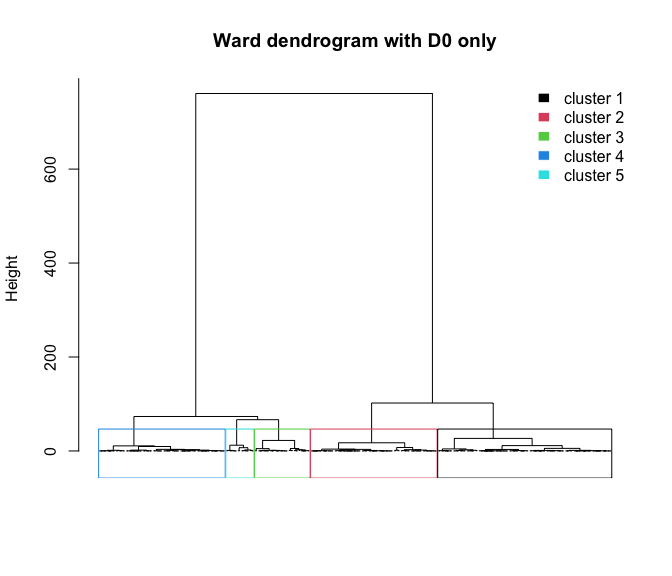This partition is plotted on the estuay map.

# cut the dendrogram to get the partition in 5 clusters
P5 <- cutree(tree,5)
city_label <- as.vector(map$"NOM_COMM") names(P5) <- city_label plot(map, border = "grey", col = P5, main = "Partition P5 obtained with D0 only") legend("topleft", legend = paste("cluster",1:5), fill = 1:5, bty = "n", border = "white")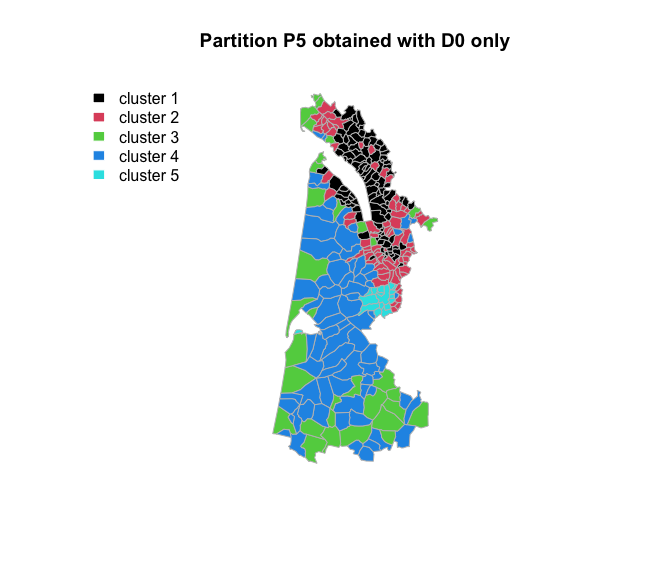This map shows that municipalities in the same cluster of the partition P5 are not necessary contiguous. For instance municipalities in cluster 5 are contiguous wehereas municipalities in cluster 3 are separated. # list of the municipalities in cluster 5 city_label[which(P5==5)] ##  "ARCACHON" "BASSENS" "BEGLES" ##  "BORDEAUX" "LE BOUSCAT" "BRUGES" ##  "CARBON-BLANC" "CENON" "EYSINES" ##  "FLOIRAC" "GRADIGNAN" "LE HAILLAN" ##  "LORMONT" "MERIGNAC" "PESSAC" ##  "TALENCE" "VILLENAVE-D'ORNON" ### Change the partition to take geographical constraint into account In order to get more spatially compact clusters, the matrix D1 of the distances between the town halls of the municipalities, is included in the clustering process along with the mixing parameter alpha used to set the importance of D0 and D1. D1 <- as.dist(D.geo) # the geographic distances between the municipalities The mixing parameter alpha is chosen in such a way that the geographical cohesion of the clusters is improved without deteriorating too much the socio-economic cohesion. #### Choice of the mixing parameter alpha The mixing parameter alpha in $$[0,1]$$ sets the importance of D0 and D1 in the clustering process. When alpha=0 the geographical dissimilarities are not taken into account and when alpha=1 it is the socio-economic distances which are not taken into account and the clusters are obtained with the geographical distances only. The idea is then to calculate separately the socio-economic homogeneity (denoted Q0) and the geographic homogneity (denoted Q1) of the partitions obtained for a range of different values of alpha and a given number of clusters $$K$$. The homogeneity Q0 (resp. Q1) is the proportion of explained inertia calculated with D0 (resp. D1). range.alpha <- seq(0,1,0.1) K <- 5 cr <- choicealpha(D0, D1, range.alpha, K, graph = FALSE) cr$Q # proportion of explained inertia
##                  Q0        Q1
## alpha=0   0.8134914 0.4033353
## alpha=0.1 0.8123718 0.3586957
## alpha=0.2 0.7558058 0.7206956
## alpha=0.3 0.7603870 0.6802037
## alpha=0.4 0.7062677 0.7860465
## alpha=0.5 0.6588582 0.8431391
## alpha=0.6 0.6726921 0.8377236
## alpha=0.7 0.6729165 0.8371600
## alpha=0.8 0.6100119 0.8514754
## alpha=0.9 0.5938617 0.8572188
## alpha=1   0.5016793 0.8726302

The plot of the curves of Q0 and Q1 is a tool to choose a value of alpha that is a compromise between the lost in socio-economic homogeneity and the gain in geographic cohesion.

?plot.choicealpha
plot(cr)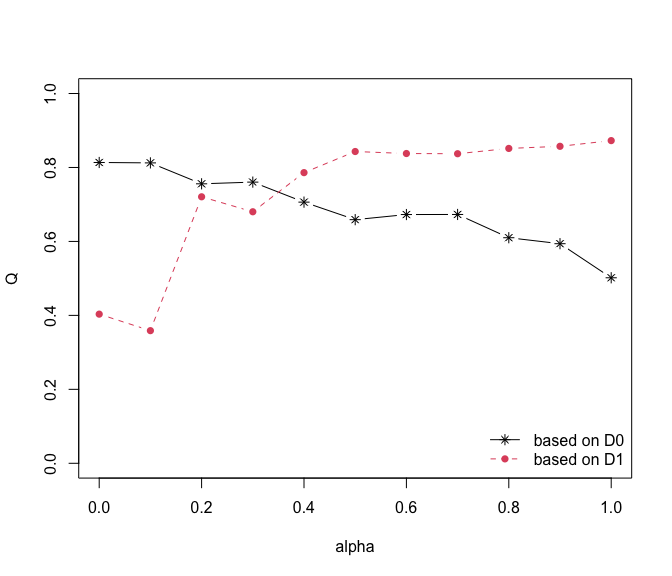We see that the proportion of explained inertia calculated with D0 (the socio-economic distances) is equal to 0.81 when alpha=0 and decreases when alpha inceases (black line). On the contrary the proportion of explained inertia calculated with D1 (the geographical distances) is equal to 0.87 when alpha=1 and decreases when alpha decreases (red line).

Here the plot suggest to choose alpha=0.2 which correponds to a lost of socio-economic homogeneity of only 7 % and a gain of geographic homogeneity of about 17 %.

#### Modified partition obtained with alpha=0.2

The dendrogram obtained with the function hclustgeo and alpha=0.2 of is cut to get the new partition in 5 clusters.

tree <- hclustgeo(D0,D1,alpha=0.2)
P5bis <- cutree(tree,5)

The modified partition P5bis is visualized on the map.

sp::plot(map, border = "grey", col = P5bis,
main = "Partition P5bis obtained with alpha=0.2
and geographical distances")
legend("topleft", legend=paste("cluster",1:5),
fill=1:5, bty="n",border="white")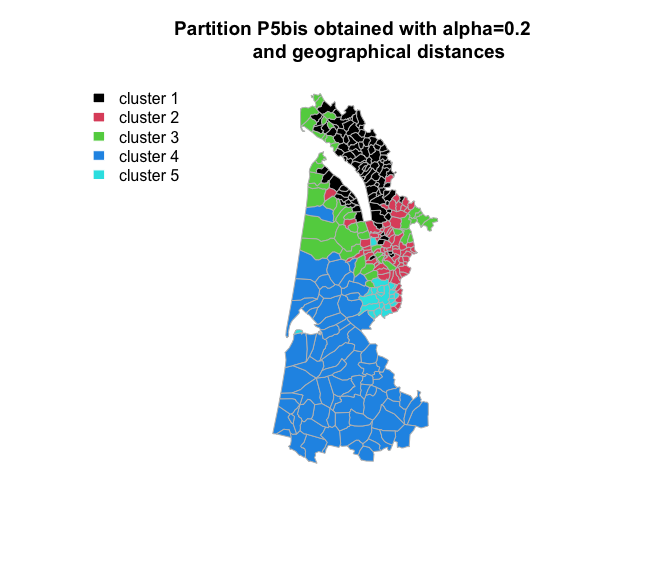We see that the geographical cohesion of the partition P5bis is increased compared to partition P5.

### Change the partition to take neighborhood constraint into account

Here a different matrix of dissimilarities D1 is considered to take the neighborhood between the municipalities into account rather than the geographical distance. Two municipalities with contiguous boundaries (sharing one or more boundary point) are considered as neighbours. The adjacency matrix A is the binary matrix of the neighbourhoods between the municipalities.

library(spdep)
?poly2nb
list.nb <- poly2nb(map, row.names = rownames(dat)) #list of neighbours of each city
?nb2mat
A <- nb2mat(list.nb,style="B")
diag(A) <- 1
colnames(A) <- rownames(A) <- city_label
A[1:5,1:5]
##          ARCES ARVERT BALANZAC BARZAN BOIS
## ARCES        1      0        0      1    0
## ARVERT       0      1        0      0    0
## BALANZAC     0      0        1      0    0
## BARZAN       1      0        0      1    0
## BOIS         0      0        0      0    1

The dissimilarity matrix D1 is then 1 minus A.

D1 <- as.dist(1-A)

#### Choice of the mixing parameter alpha

The procedure for the choice of alpha is repeated here with the new matrix D1.

range.alpha <- seq(0,1,0.1)
K <- 5
cr <- choicealpha(D0, D1, range.alpha,
K, graph=FALSE)
plot(cr)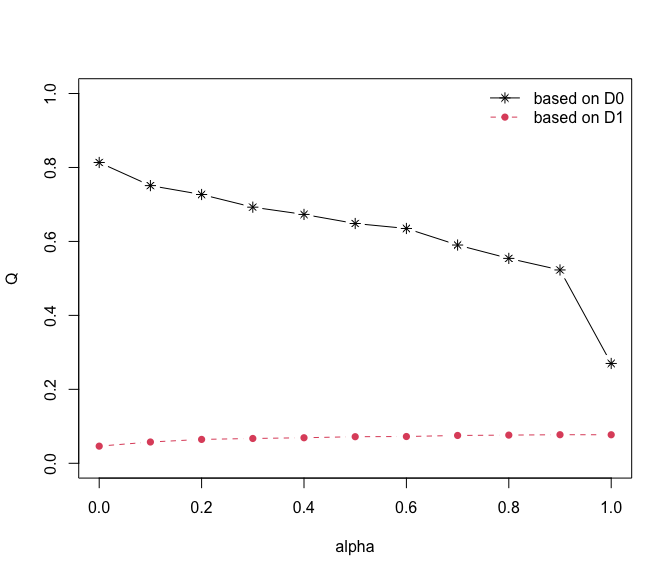The explained inertia calculated here with D1 (red curve) is much smaller than the explained inertia calculated with D0 (black curve). To overcome this problem, the normalized proportion of explained inertia (Qnorm) is plotted.

cr\$Qnorm # normalized proportion of explained inertia
##              Q0norm    Q1norm
## alpha=0   1.0000000 0.6009518
## alpha=0.1 0.9231102 0.7462157
## alpha=0.2 0.8935510 0.8370184
## alpha=0.3 0.8514257 0.8698485
## alpha=0.4 0.8272982 0.8952084
## alpha=0.5 0.7971311 0.9320703
## alpha=0.6 0.7806226 0.9386452
## alpha=0.7 0.7255676 0.9756566
## alpha=0.8 0.6811587 0.9880916
## alpha=0.9 0.6427471 1.0018473
## alpha=1   0.3318727 1.0000000
plot(cr, norm = TRUE)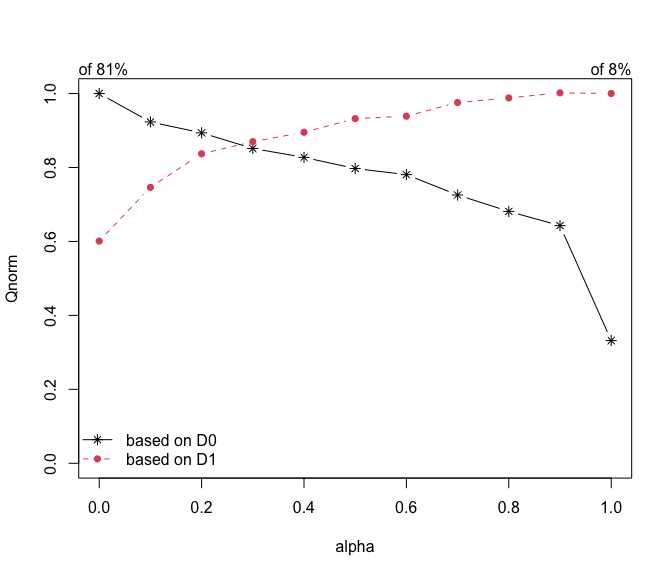With D0 the curve starts from 100% and decreases as alpha increases from 0 to 1. With D1 the curve starts from 100% (on the right) and decreases as alpha decreases from 1 to 0. This plot suggests to choose alpha=0.2

#### Modified partition obtained with alpha=0.2

The dendrogram obtained with the function hclustgeo and alpha=0.2 of is cut to get a new partition in 5 clusters.

tree <- hclustgeo(D0, D1, alpha  =0.2)
P5ter <- cutree(tree,5)
sp::plot(map, border="grey", col=P5ter,
main=" Partition P5ter obtained with
alpha=0.2 and neighborhood dissimilarities")
legend("topleft", legend=1:5, fill=1:5, col=P5ter)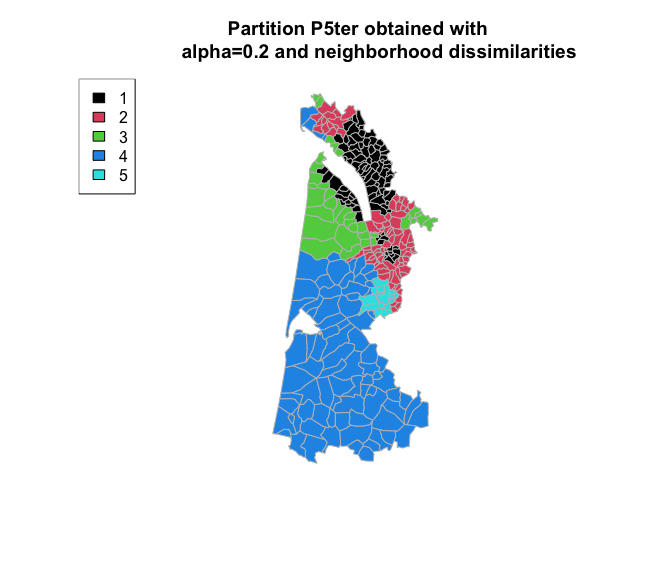This partition P5ter is spatially more compact than P5bis. This is not surprising since dissimilarities are build from the adjacency matrix which gives more importance local neighborhoods. However since the clustering process is based on soft contiguity constraints, municipalities that are not neighbors are allowed to be in the same clusters. This is the case for instance for cluster 4 where some municipalities are located in the north of the estuary whereas most are located in the southern area (corresponding to forest areas).

##Reference M. Chavent, V. Kuentz-Simonet, A. Labenne, J. Saracco. ClustGeo: an R package for hierarchical clustering with spatial constraints.Comput Stat (2018) 33: 1799-1822.